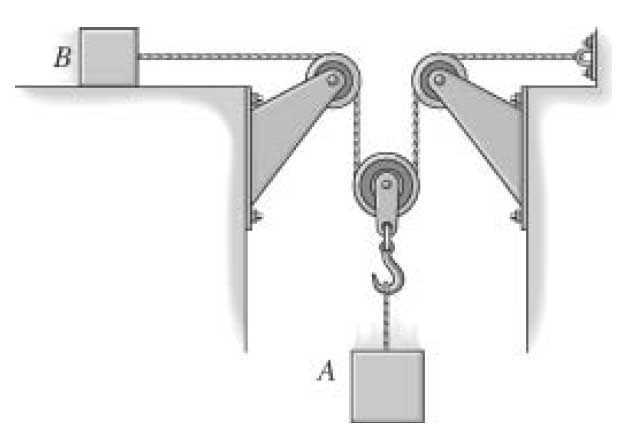# Force and Acceleration

## Homework Statement

At a given instant block A of weight 10-lb is moving downward with speed 6 ft/s. Determine its speed at a later time t = 2s. Block B has a weight 4lb and the coefficient of kinetic friction between it and the horizontal plane is μ = 0.2. Neglect the mass of the pulleys and cord.T = 3.385 $$a_{A}$$ = 10.403 $$ft/s^{2}$$ $$a_{B}$$ = - 20.806 $$ft/s^{2}$$

not sure if these are correct seeing i get different answers.

## Homework Equations

T - $$\mu_{k}w_{B}$$ = $$\frac{-w_{B}}{g}a_{B}$$

2T - $$w_{A} = (\frac{-w_{A}}{g}) (-a_{A})$$

$$2a_{A} + a_{B} = 0$$

## The Attempt at a Solution

since $$2a_{A} + a_{B} = 0$$ i'd solve for $$a_{A}$$ and $$a_{B}$$ in the first 2 equations and sub into the third one

$$2(\frac{2Tg}{w_{A}} - g) + (\frac{-Tg}{w_{B}} + \mu_{k}g)$$ = 0

then i solve for T

$$\frac{2g - \mu_{k}g}{g(\frac{4}{w_{A}} - \frac{1}{w_{B}})}$$ = T

T= 12N

then sub back into one of the first 2 equations to solve for either $$a_{A}$$ or $$a_{B}$$.

$$a_{B}$$ = -90.16$$ft/s^{2}$$
$$a_{A}$$ = 45.08$$ft/s^{2}$$

any help would b nice
cheers,

Last edited:

LowlyPion
Homework Helper
ft/sec

lbs

m/s

m/s2

Unit potpourri is never a good recipe.

ft/sec

lbs

m/s

m/s2

Unit potpourri is never a good recipe.
it was late...i changed the units, i was just used to $$m/s^{2}$$ from previous physics courses....